×

Search anything:

# Swapping a queue in C++ STL

#### Software Engineering C++Reading time: 30 minutes | Coding time: 5 minutes

Queue is an abstract data type that follows the First In First Out(FIFO) order. The C++ STL has a container for queue. In this article we are going to discuss about swap member function of the queue container.

Swapping two queues is only possible when both the queues are of same type but the size of both the queues need not be the same.

### Consider the following example of swapping two queues

Let queue1 contain 10, 20, 30 and queue2 contain 11, 22, 33.

After swapping queue1 contains 11, 22, 33 and queue2 contains 10, 20, 30 (refer the image below).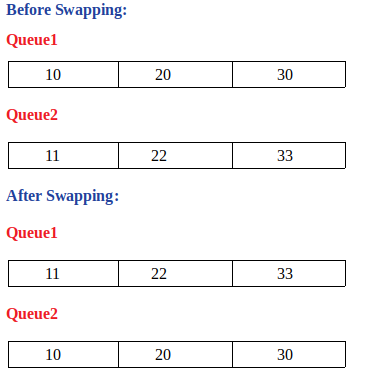### We can swap queues in two ways:

• using std::queue::swap
• using std::swap

## std::queue::swap

``````void swap (queue& q) noexcept();
``````
• Exchanges the contents of the current container adaptor (this) by those of q
• The queue::swap() internally calls the non-member function std::swap() which is also illustrated in this article

### Illustration of queue::swap()

Let queue1 contain 1, 2, 3 and queue2 contain 4, 5, 6, 7. After swapping >queue1 contains 4, 5, 6, 7 and queue2 contains 1, 2, 3 (refer the image below).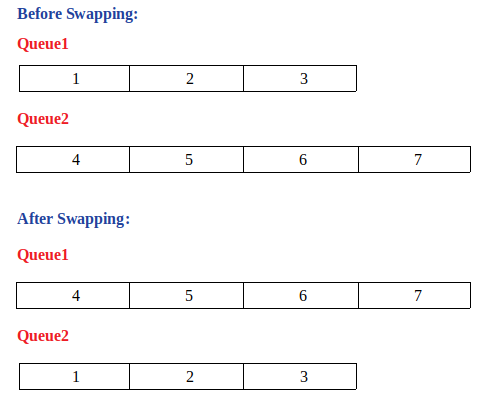``````// C++ program to illustrate std::queue::swap()

#include <iostream>
#include <queue>

using namespace std;

void printQ(queue <int> q); // prints all the elements of a queue

int main()
{
queue <int> q1, q2;
//initalising  queue1
q1.push(1);
q1.push(2);
q1.push(3);

//initialising queue2
q2.push(4);
q2.push(5);
q2.push(6);
q2.push(7);

cout<<"Before Swapping:\n";
cout<<"Queue1: ";
printQ(q1);
cout<<"Queue2: ";
printQ(q2);

q1.swap(q2); //swap queues q1 and q2

cout<<"\nAfter Swapping:\n";
cout<<"Queue1: ";
printQ(q1);
cout<<"Queue2: ";
printQ(q2);
}

void printQ(queue <int> q)
{
while(!q.empty())    //loop until queue is empty
{
cout<<q.front()<<" "; // print each element
q.pop();
}
cout<<"\n";
}
``````

Output:

``````Before Swapping:
Queue1: 1 2 3
Queue2: 4 5 6 7

After Swapping:
Queue1: 4 5 6 7
Queue2: 1 2 3
``````

Note: queue::swap() is introduced in C++11. Earlier compilers might not support it.

## std::swap

``````template <class T, class Container>
void swap (queue<T,Container>& x, queue<T,Container>& y) noexcept(noexcept(x.swap(y)));
``````
• Exchanges the contents of x and y.
• This is the non-member function that is called internally by std::queue::swap()

### Illustration of std::swap() on queues

Let queue1 contain a, b, c, d and queue2 contain e, f, g. After swapping queue1 contains e, f, g and queue2 contains a, b, c, d (refer the image below)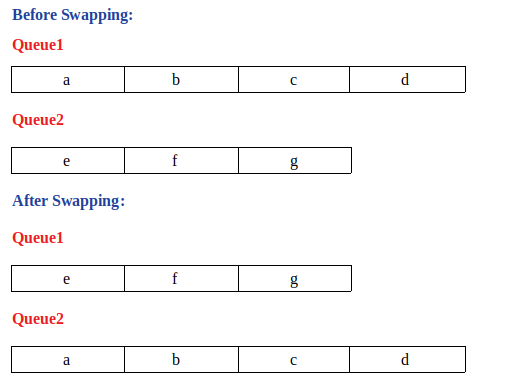``````// C++ program to illustrate std::swap() on queues

#include <iostream>
#include <queue>

using namespace std;

void printQ(queue <char> q); // prints all the elements of a queue

int main()
{
queue <char> q1, q2;
// initialise queue1
q1.push('a');
q1.push('b');
q1.push('c');
q1.push('d');

//initialise queue2
q2.push('e');
q2.push('f');
q2.push('g');

cout<<"Before Swapping:\n";
cout<<"Queue1: ";
printQ(q1);
cout<<"Queue2: ";
printQ(q2);

swap(q1, q2); //swap queues q1 and q2

cout<<"\nAfter Swapping:\n";
cout<<"Queue1: ";
printQ(q1);
cout<<"Queue2: ";
printQ(q2);
}

void printQ(queue <char> q)
{
while(!q.empty()) //loop until queue is not empty
{
cout<<q.front()<<" "; // print each element
q.pop();
}
cout<<"\n";
}
``````

Output:

``````Before Swapping:
Queue1: a b c d
Queue2: e f g

After Swapping:
Queue1: e f g
Queue2: a b c d
``````

## Errors and Exceptions

• Throws an error if both queues are not of the same type.
• Otherwise, it has a basic no exception guarantee.

## Return Value

• void
``````#include <typeinfo> // include this header to know the type

cout<<"Type: "<<typeid(q1.swap(q2)).name()<<"\n";
cout<<"Type: "<<typeid(swap(q1,q2)).name()<<"\n";
``````

Output:

``````Type: v
Type: v
``````

• Constant

## Swapping queues that contain other containers and user defined objects

• Queues containing user defined objects and other containers can also be swapped

### Illustration of std::queue::swap() on queues that contain pair

Let queue1 contain (1,a), (2,b), (3,c) and queue2 contain (4,d), (5,e), (6,f). After swapping queue1 contains (4,d), (5,e), (6,f) and queue2 contains (1,a), (2,b), (3,c) (refer the image below).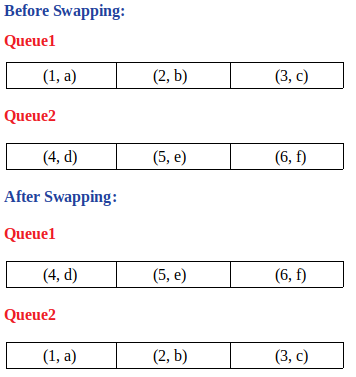``````//C++ program to illustrate swapping queues of pairs
#include <iostream>
#include <queue>
#include <utility> //include this header to use pair

using namespace std;

void printQ(queue<pair<int, char>> q); // prints all the elements of the queue

int main()
{
queue <pair<int, char>> q1, q2;
//initialise queue1
q1.push(make_pair(1,'a'));
q1.push(make_pair(2,'b'));
q1.push(make_pair(3,'c'));

//initialise queue2
q2.push(make_pair(4,'d'));
q2.push(make_pair(5,'e'));
q2.push(make_pair(6,'f'));

cout<<"Before Swapping:\n";
cout<<"Queue1:\n";
printQ(q1);
cout<<"Queue2:\n";
printQ(q2);

q1.swap(q2); //swap q1 and q2

cout<<"\nAfter Swapping:\n";
cout<<"Queue1:\n";
printQ(q1);
cout<<"Queue2:\n";
printQ(q2);

return 0;

}

void printQ(queue<pair<int, char>> q)
{
while(!q.empty()) //loop until queue is empty
{
cout<<q.front().first<<" - "<<q.front().second<<"\n"; //print contents of each element
q.pop();
}
}
``````

Output:

``````Before Swapping:
Queue1:
1 - a
2 - b
3 - c
Queue2:
4 - d
5 - e
6 - f

After Swapping:
Queue1:
4 - d
5 - e
6 - f
Queue2:
1 - a
2 - b
3 - c
``````

### Illustration of std::swap() on queues contianing user defined objects

Let us assume a student structure that has three fields i.e., roll, name, percent. let queue1 contain (1, abc, 95.5), (2, def, 96.0), (3, ghi, 96.5) and queue2 contain (4, jkl, 97.0), (5, mno, 97.5), (6, pqr, 98.0), (7, stu, 98.5). After swapping queue1 contains (4, jkl, 97.0), (5, mno, 97.5), (6, pqr, 98.0), (7, stu, 98.5) and queue2 contains (1, abc, 95.5), (2, def, 96.0), (3, ghi, 96.5)(refer the image below).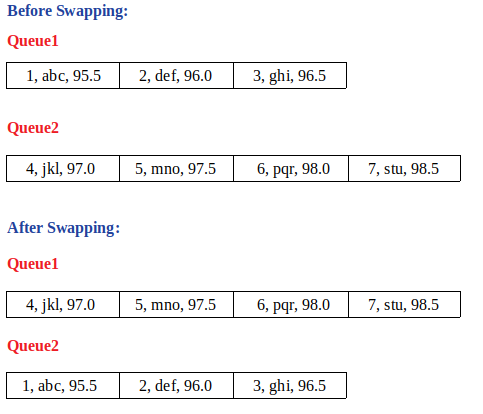``````//C++ program to illustrate swapping of queues containing user defined objects
#include <iostream>
#include <queue>
#include <string>
#include <iomanip>

using namespace std;

struct Student // user defined structure
{
int roll;
string name;
float percent;
};

typedef struct Student st; //giving a short name for struct Student

st* newStudent(int, string, float); // initialises a new student object
void printQ(queue <st>); // prints all the queue elements

int main()
{
queue <st> q1,q2;
// initialise queue1
q1.push(*newStudent(1,"abc",95.5));
q1.push(*newStudent(2,"def",96.0));
q1.push(*newStudent(3,"ghi",96.5));

// initialise queue2
q2.push(*newStudent(4,"jkl",97.0));
q2.push(*newStudent(5,"mno",97.5));
q2.push(*newStudent(6,"pqr",98.0));
q2.push(*newStudent(7,"stu",98.5));

cout<<"Before Swapping:\n";
cout<<"Queue1:\n";
printQ(q1);
cout<<"Queue2:\n";
printQ(q2);

swap(q1,q2); // swap q1 and q2

cout<<"\nAfter Swapping:\n";
cout<<"Queue1:\n";
printQ(q1);
cout<<"Queue2:\n";
printQ(q2);

return 0;
}

st* newStudent(int roll, string name, float percent)
{
st *student = new st; // create a new Student object and assign values to it
student->roll = roll;
student->name = name;
student->percent = percent;

return student; // return the student object
}

void printQ(queue <st> q)
{
while(!q.empty()) // loop until queue is empty
{
cout<<setprecision(2)<<fixed<<q.front().roll<<" - "<<q.front().name<<" - "<<q.front().percent<<'\n'; // print contents of each element
q.pop();
}
}
``````

Output:

``````Before Swapping:
Queue1:
1 - abc - 95.50
2 - def - 96.00
3 - ghi - 96.50
Queue2:
4 - jkl - 97.00
5 - mno - 97.50
6 - pqr - 98.00
7 - stu - 98.50

After Swapping:
Queue1:
4 - jkl - 97.00
5 - mno - 97.50
6 - pqr - 98.00
7 - stu - 98.50
Queue2:
1 - abc - 95.50
2 - def - 96.00
3 - ghi - 96.50
``````